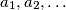### IMO Shortlist 2005 problem N2

Kvaliteta:
Avg: 4,0
Težina:
Avg: 6,0
Let$a_1,a_2,\ldots$ be a sequence of integers with infinitely many positive and negative terms. Suppose that for every positive integer$n$ the numbers$a_1,a_2,\ldots,a_n$ leave$n$ different remainders upon division by$n$.

Prove that every integer occurs exactly once in the sequence$a_1,a_2,\ldots$.
Izvor: Međunarodna matematička olimpijada, shortlist 2005